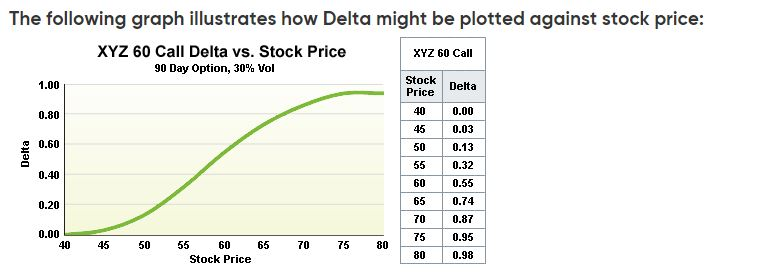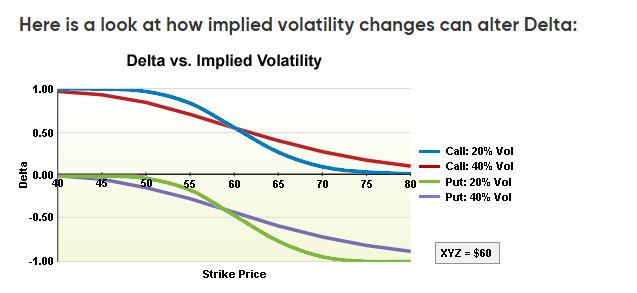Back
BlogsDelta

FED'S BULLARD: AT THIS POINT, I SEE POLICY RATE IN THE RANGE OF 5.25% TO 5.5% AS APPROPRIATEGamma

# Delta

Categories

Learning

The GreeksDELTA Definition: The rate of change in the theoretical value of an option over a one-point change in underlying price. Expressed as a percentage, it can also be interpreted as the equivalent amount of underlying that an option represents, or hedge ratio. Calls have positive deltas, puts have negative deltas.

--The Delta has various interpretations: I. The change in the price of the option in response to the change in the price of the underlying contract. A positive delta means that the option's price is positively correlated with the underlying changes (as in the case of a call), and a negative delta means that the option's price is negatively correlated with the underlying changes (as in the case of a put).Ex: The 100 call is trading for 3.00 and has a +50 delta (with the underlying stock trading at 100) If the price of the stock goes to 101, the call value would increase to 3.50.

II. The equivalent amount of underlying position that the option . represents also used as the effective hedge ratio.

III. Ex: The 100 put has a -50 delta, which means that your exposure in the marketplace is the equivalent of being short (negative delta) .50 or 50% of an underlying contract (in the case of equities, since 100 shares is considered one underlying it is equivalent to being short 50 shares.) If you bought one of these puts and wanted to hedge that exposure, you would need to buy 50 shares in order to delta neutralize your positions. The probability that the option will be ITM or exercised at expiration. (If we ignore the sign of the delta.) Ex: The 100 call, with the stock trading at 100, would have an approximately.50 delta-the call is exactly ATM and has a 50% chance of going in the money.

IV. The delta of the call and the put at the same strike should add up to approximately 1.00 (without taking into consideration the sign of the delta-long or short). Ex: The 100 call, with the stock trading at 100, would have an approximately .50 delta-the call is exactly ATM and has a 50% chance of going in the money. By the same token, the ATM put would also have a.50 delta, or a 50% chance of ending up in the money. Together, the two deltas add up to a 100% probability that one or the other will be ITM at expiration.## QUIZ TIME

Test your skills with are questionnaire and challenge the waves.

If an option has a 30% delta and the stock/underlying moves .50 , what would be the closest expected change in the option price?

The GreeksDarren Krett

Friday, 10 February 2023

## Delta Neutrality

This just means that they have various positions on (some bullish and some bearish) but their overall portfolio is pretty delta neutral. It
Delta Neutrality

0